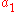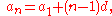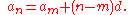xArithmetic progressionOverview

In mathematics
Mathematics
Mathematics is the study of quantity, space, structure, and change. Mathematicians seek out patterns and formulate new conjectures. Mathematicians resolve the truth or falsity of conjectures by mathematical proofs, which are arguments sufficient to convince other mathematicians of their validity...

, an arithmetic progression (AP) or arithmetic sequence is a sequence
Sequence
In mathematics, a sequence is an ordered list of objects . Like a set, it contains members , and the number of terms is called the length of the sequence. Unlike a set, order matters, and exactly the same elements can appear multiple times at different positions in the sequence...

of number
Number
A number is a mathematical object used to count and measure. In mathematics, the definition of number has been extended over the years to include such numbers as zero, negative numbers, rational numbers, irrational numbers, and complex numbers....

s such that the difference between the consecutive terms is constant. For instance, the sequence 3, 5, 7, 9, 11, 13, … is an arithmetic progression with common difference 2.

If the initial term of an arithmetic progression isand the common difference of successive members is d, then the nth term of the sequence is given by:and in generalA finite portion of an arithmetic progression is called a finite arithmetic progression and sometimes just called an arithmetic progression.

The behavior of the arithmetic progression depends on the common difference d.